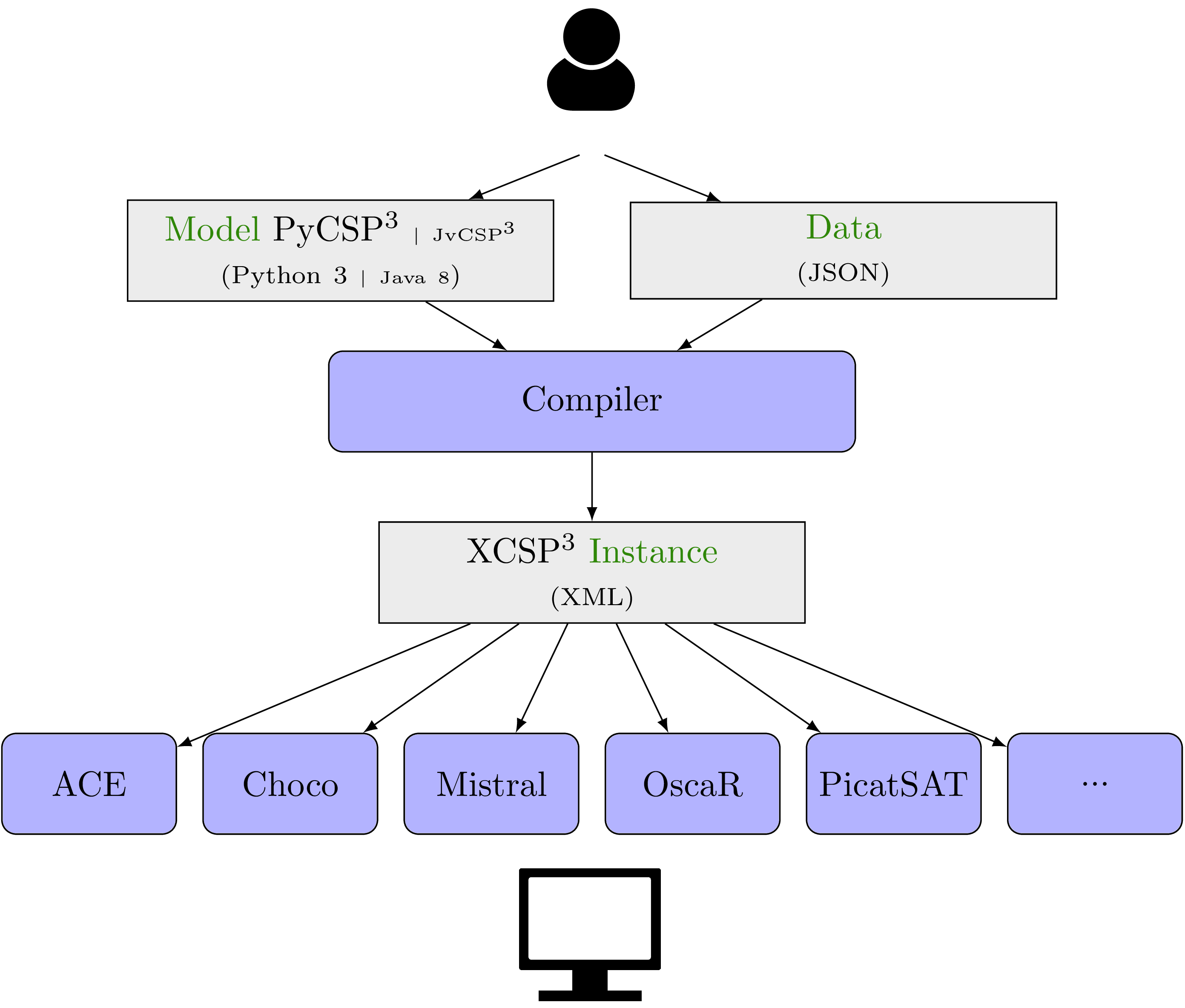PyCSP3

# PyCSP3

PyCSP3 is a Python library that allows us to write models of combinatorial constrained problems in a declarative manner. Currently, with PyCSP3, you can write models of constraint satisfaction and optimization problems. More specifically, you can build models for:

• CSP (Constraint Satisfaction Problem)
• COP (Constraint Optimization Problem)

In this website, you will find all that you need to know about Constraint Programming (CP) and PyCSP3, with more than 50 Jupyter Notebooks:

You can also read this guide .

As an illustration, below, you can find two simple examples of PyCSP3 models:

#### AllInterval Problem


from pycsp3 import *

n = data or 8

# x[i] is the ith note of the series
x = VarArray(size=n, dom=range(n))

satisfy(
# notes must occur once, and so form a permutation
AllDifferent(x),

# intervals between neighbouring notes must form a permutation
AllDifferent(abs(x[i] - x[i + 1]) for i in range(n - 1)),

# tag(symmetry-breaking)
x < x[n - 1]
)



#### Golomb Ruler Problem


from pycsp3 import *

n = data or 10

# x[i] is the position of the ith tick
x = VarArray(size=n, dom=range(n * n))

satisfy(
# all distances are different
AllDifferent(abs(x[i] - x[j]) for i, j in combinations(range(n), 2)),

# tag(symmetry-breaking)
[x == 0, Increasing(x, strict=True)]
)

minimize(
# minimizing the position of the rightmost tick
x[-1]
)


### Last News

August 2022
Forthcoming: XCSP3 Competition, held with FLoC 2022; the call is available here. Deadline 23th of May, 2022.
July 2022
Forthcoming: PyCSP3 2.1, with new version of embedded solvers (ACE and Choco) and additional constraints (Precedence, BinPacking, Knapsack, Flow, MinimumArg, MaximumArg).
December 15, 2021
PyCSP3 2.0 , Version 2 of the Python library PyCSP3.
December 15, 2021
ACE 2.0 , Version 2 of the generic constraint solver ACE (written in Java).
January 16, 2021
Specifications of XCSP3, Version 3.0.7

## Requirements

• For building and compiling models, you need:
• Python 3.6 (at least) to be installed
• Python package PyCSP3 to be installed, for example from PyPi: pip install pycsp3
• For running the embedded solvers (ACE and Choco), you need Java 11 to be installed

Importantly, there is a complete separation between the modeling and solving phases: you write a model, you compile it (while providing some data) in order to generate an XCSP3 instance (file), and you solve that problem instance by means of a constraint solver. You can also directly pilot the solving procedure in PyCSP3 possibly conducting an incremental solving strategy.

In a nutshell, the main ingredients of the complete tool chain we propose for handling combinatorial constrained problems are:

• PyCSP3: a Python library for modelling constrained problems, which is described in this document (or equivalently, JvCSP3, a Java-based API)
• XCSP3: an intermediate format used to represent problem instances while preserving structure of models

A shown in the figure below, the user who wishes to solve a combinatorial constrained problem has to:

• write a model using the Python library PyCSP3 (i.e., write a Python file), or the Java modeling API JvCSP3 (i.e., write a Java file)
• provide a data file (in JSON format) for a specific problem instance to be solved
• compile both files (model and data) so as to generate an XCSP3 instance (file)
• solve the XCSP3 file (problem instance under format XCSP3) by using a constraint solver as, e.g., ACE, Choco, OscaR or PicatSAT